# What is Power Factor & Power Triangle

## Power Factor:

Power Factor is defined as the cosine of an angle between the current and voltage. Otherwise, the ratio between the real power to reactive power is called power factor.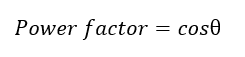Unit: Since power factor is a ratio quantity, that’s why it does not have any units.

In generally power is the ability to do the work. In the electrical domain, electrical power is the amount of electrical energy that can be transferred to some other form (heat, light etc.) per unit time.

Mathematically it is the product of voltage drop across the element and current flowing through the element is called power.

Considering first the DC circuits, having only DC voltage sources (any current source or voltage source), the inductors behave as short circuit and capacitors behave like an open circuit in steady state.

Hence the entire circuit behaves like a resistive circuit and the entire electrical power is dissipated in the form of heat. Here the voltage and current are in the same phase and the total electrical power is given by.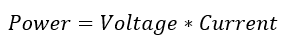But in AC power system due to the alternating nature (the frequency), inductor and capacitor provide a certain impedance to the circuit. An inductive circuit store the energy in the form of a magnetic field.

The capacitor stores the energy in the form of the electric field. But in resister works same like DC circuits. Due to the inductive and capacitive action, the power factor presents.

## Power Triangle: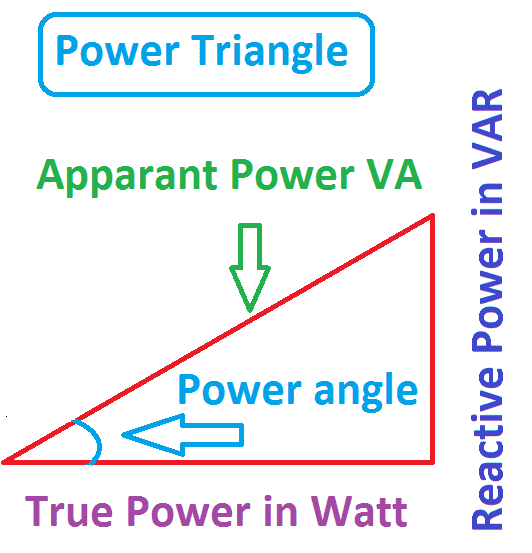The relationships between real power P, reactive power Q, apparent power VI and the power angle θ can be represented by the power triangle. The power triangle is shown in Figure for an inductive load and capacitive load in which case θ and Q are positive and Q are negative.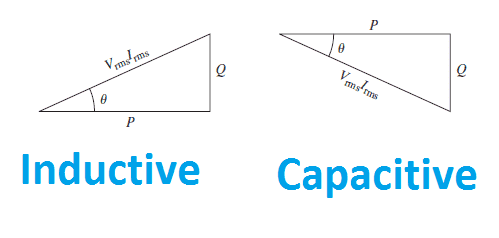Generally, The power factor is equal to the real or true power P in watts (W) divided by the apparent power |S| in volt-ampere (VA) from the Power triangle: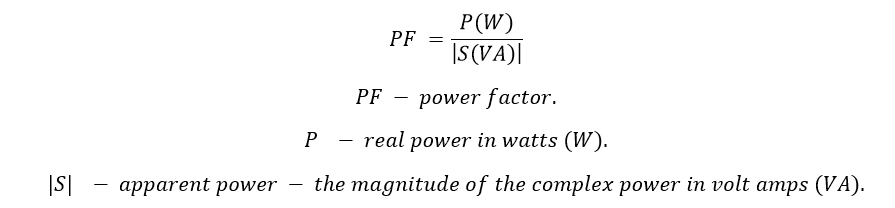Generally, Power factor tells about the phase relationship between the voltage and current. Under ideal conditions current and voltage are “in phase” and the power factor is “100%.” If inductive loads (motors) are present, power factor less than 100% (typically 80 to 90%) can occur.

Low power factor, electrically speaking, causes heavier current to flow in power distribution lines in order to deliver a given number of kilowatts to an electrical load. Due to this, we have to spend unnecessary energy cost as power loss and installation cost as increased in equipment capacity.

### The power factor for the resistive circuit:

Consider the Power Triangle. For the purely resistive circuit, the power angle is equal to zero because of the voltage and current will be in phase; hence the power factor is equal to 1 (Cos0=1), because the reactive power equals zero. Here, the power triangle would look like a horizontal line, because the opposite (reactive power) side would have zero length.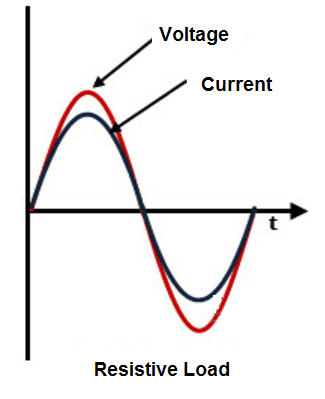### Pure Inductive Circuits:

For the purely inductive circuit which means the circuit should not have the internal resistance, hence the current lags the voltage by 90 deg. therefore the power factor is zero (Cos 90=0), because true power equals zero. Here, the power triangle would look like a vertical line, because the adjacent (true power) side would have zero length.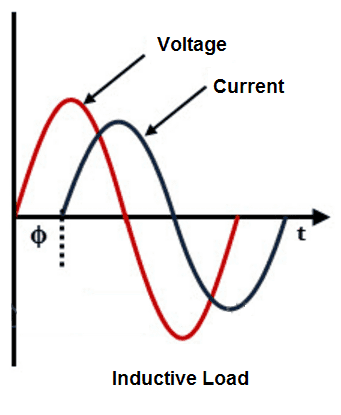### Pure capacitive Circuits:

The same could be said for a purely capacitive circuit. If there are no dissipative (resistive) components in the circuit, then the true power must be equal to zero, making any power in the circuit purely reactive. The power triangle for a purely capacitive circuit would again be a vertical line.

Also in Pure Capacitive circuit, the current leads the voltage by 90 deg. Which means cos-90=-1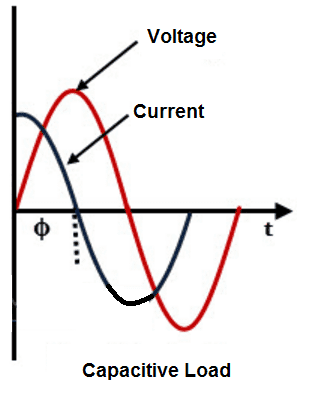## Key points:

• The cosine of angle between the voltage and current is called power factor cosϕ
• In an inductive circuit, the power factor in lagging nature